# NCERT Solution (Miscellaneous) - Relations and Functions JEE Notes | EduRev

## JEE : NCERT Solution (Miscellaneous) - Relations and Functions JEE Notes | EduRev

The document NCERT Solution (Miscellaneous) - Relations and Functions JEE Notes | EduRev is a part of the JEE Course Mathematics (Maths) Class 11.
All you need of JEE at this link: JEE

Ques 1: The relation f is defined by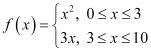The relation g is defined by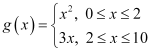Show that f is a function and g is not a function.
Ans:  The relation f is defined asIt is observed that for

0 ≤ x < 3, f(x) = x2

3 < x ≤ 10, f(x) = 3x

Also, at x = 3, f(x) = 32 = 9 or f(x) = 3 × 3 = 9

i.e., at x = 3, f(x) = 9

Therefore, for 0 ≤ x ≤ 10, the images of f(x) are unique.

Thus, the given relation is a function.

The relation g is defined asIt can be observed that for x = 2, g(x) = 22 = 4 and g(x) = 3 × 2 = 6

Hence, element 2 of the domain of the relation g corresponds to two different images i.e., 4 and 6. Hence, this relation is not a function.

Ques 2: If f(x) = x2, find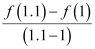.
Ans: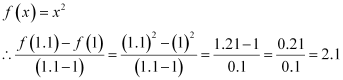Ques 3: Find the domain of the function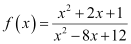Ans:  The given function is.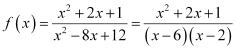It can be seen that function f is defined for all real numbers except at x = 6 and x = 2.
Hence, the domain of f is R – {2, 6}.

Ques 4: Find the domain and the range of the real function f defined by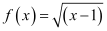.
Ans:   The given real function is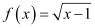.
It can be seen that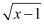is defined for (x – 1) ≥ 0.
i.e.,is defined for x ≥ 1.
Therefore, the domain of f is the set of all real numbers greater than or equal to 1 i.e., the domain of f = [1,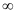).
As x ≥ 1 ⇒ (x – 1) ≥ 0 ⇒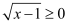Therefore, the range of f is the set of all real numbers greater than or equal to 0 i.e., the range of f = [0,).

Ques 5: Find the domain and the range of the real function f defined by f (x) = |x – 1|.
Ans:  The given real function is f (x) = |x – 1|.
It is clear that |x – 1| is defined for all real numbers.
∴Domain of f = R
Also, for xR, |x – 1| assumes all real numbers.
Hence, the range of f is the set of all non-negative real numbers.

Ques 6: Let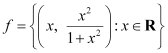be a function from R into R. Determine the range of f.
Ans: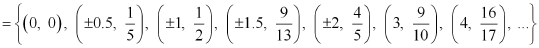The range of f is the set of all second elements. It can be observed that all these elements are greater than or equal to 0 but less than 1.
[Denominator is greater numerator]
Thus, range of f = [0, 1)

Ques 7: Let f, g: R → R be defined, respectively by f(x) = x  +1, g(x) = 2x – 3. Find f +  g, fg and f/g.
Ans:   f, g: RR is defined as f(x) = x + 1, g(x) = 2x – 3

(f  + g) (x) = f(x) + g(x) = (x  +1) (2x – 3) = 3x – 2

∴(f+ g) (x) = 3x – 2

(f – g) (x) = f(x) – g(x) = (x + 1) – (2x – 3) = x + 1 – 2x + 3 = – x + 4

∴ (f – g) (x) = –x + 4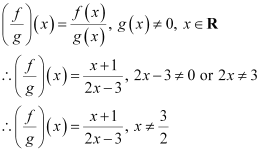Ques 8: Let f = {(1, 1), (2, 3), (0, –1), (–1, –3)} be a function from Z to Z defined by f(x) = ax +  b, for some integers a, b. Determine a, b.
Ans:  f = {(1, 1), (2, 3), (0, –1), (–1, –3)}

f(x) = ax +  b

(1, 1) ∈ f

f(1) = 1

a × 1 + b = 1

a  + b = 1

(0, –1) ∈ f

f(0) = –1

a × 0 + b = –1

b = –1

On substituting b = –1 in a  + b = 1, we obtain a  (–1) = 1 ⇒ a = 1 + 1 = 2.

Thus, the respective values of a and b are 2 and –1.

Ques 9: Let R be a relation from N to N defined by R = {(a, b): a, b ∈ N and a = b2}. Are the following true?
(i) (a, a) ∈ R, for all a ∈ N
(ii) (a, b) ∈ R, implies (b, a) ∈ R
(iii) (a, b) ∈ R, (b, c) ∈ R implies (a, c) ∈ R.
Ans:  R = {(a, b): a, bN and a = b2}

(i) It can be seen that 2 ∈ N;however, 2 ≠ 22 = 4.

Therefore, the statement “(a, a) ∈ R, for all aN” is not true.

(ii) It can be seen that (9, 3) ∈ N because 9, 3 ∈ N and 9 = 32.

Now, 3 ≠ 92 = 81; therefore, (3, 9) ∉ N

Therefore, the statement “(a, b) ∈ R, implies (b, a) ∈ R” is not true.

(iii) It can be seen that (9, 3) ∈ R, (16, 4) ∈ R because 9, 3, 16, 4 ∈ N and 9 = 32 and 16 = 42.

Now, 9 ≠ 42 = 16; therefore, (9, 4) ∉ N

Therefore, the statement “(a, b) ∈ R, (b, c) ∈ R implies (a, c) ∈ R” is not true.

Ques 10: Let A = {1, 2, 3, 4}, B = {1, 5, 9, 11, 15, 16} and f = {(1, 5), (2, 9), (3, 1), (4, 5), (2, 11)}. Are the following true?
(i) f is a relation from A to B
(ii) f is a function from A to B.
Ans:  A = {1, 2, 3, 4} and B = {1, 5, 9, 11, 15, 16}

∴A × B = {(1, 1), (1, 5), (1, 9), (1, 11), (1, 15), (1, 16), (2, 1), (2, 5), (2, 9), (2, 11), (2, 15), (2, 16), (3, 1), (3, 5), (3, 9), (3, 11), (3, 15), (3, 16), (4, 1), (4, 5), (4, 9), (4, 11), (4, 15), (4, 16)}

It is given that f = {(1, 5), (2, 9), (3, 1), (4, 5), (2, 11)}

(i) A relation from a non-empty set A to a non-empty set B is a subset of the Cartesian product A × B.

It is observed that f is a subset of A × B.

Thus, f is a relation from A to B.

(ii) Since the same first element i.e., 2 corresponds to two different images i.e., 9 and 11, relation f is not a function.

Ques 11: Let f be the subset of Z × Z defined by f = {(ab, a  + b): a, b ∈ Z}. Is f a function from Z to Z: justify your answer.
Ans:  The relation f is defined as f = {(ab, a +  b): a, b ∈  Z}
We know that a relation f from a set A to a set B is said to be a function if every element of set A has unique images in set B.
Since 2, 6, –2, –6 ∈ Z, (2 × 6, 2 + 6), (–2 × –6, –2 + (–6)) ∈ f
i.e., (12, 8), (12, –8) ∈ f
It can be seen that the same first element i.e., 12 corresponds to two different images i.e., 8 and –8. Thus, relation f is not a function.

Ques 12: Let A = {9, 10, 11, 12, 13} and let f: A → N be defined by f(n) = the highest prime factor of n. Find the range of f.
Ans:       A = {9, 10, 11, 12, 13}
f: A → N is defined as
f(n) = The highest prime factor of n
Prime factor of 9 = 3
Prime factors of 10 = 2, 5
Prime factor of 11 = 11
Prime factors of 12 = 2, 3
Prime factor of 13 = 13
f(9) = The highest prime factor of 9 = 3
f(10) = The highest prime factor of 10 = 5
f(11) = The highest prime factor of 11 = 11
f(12) = The highest prime factor of 12 = 3
f(13) = The highest prime factor of 13 = 13
The range of f is the set of all f(n), where n ∈ A.
∴Range of f = {3, 5, 11, 13}

Offer running on EduRev: Apply code STAYHOME200 to get INR 200 off on our premium plan EduRev Infinity!

## Mathematics (Maths) Class 11

158 videos|179 docs|161 tests

,

,

,

,

,

,

,

,

,

,

,

,

,

,

,

,

,

,

,

,

,

;